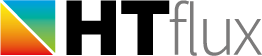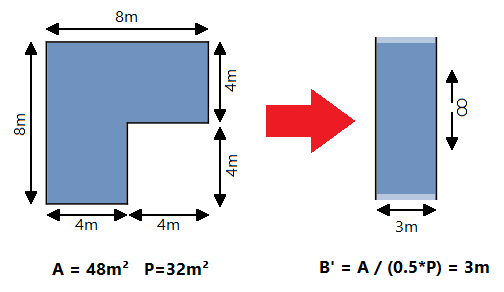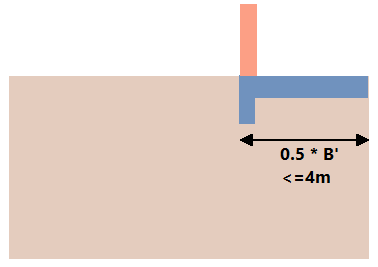# Characteristic dimension of the floor slab (B’)

The characteristic dimension of the floor is a useful concept and defined in the ISO standard 13770. The actual heat loss of a floor slab would require a three dimensional simulation. However by using the characteristic slab dimension (B’) it is possible to map this three-dimensional problem into a two-dimensional model. This mapping is an approximation of course, however it is sufficiently precise to justify its use.
So before doing ground contact calculations you should calculate this B’ value. To do so you will just have to divide the total surface of the floor slab by the half of the perimeter of the floor slab:The heat-flux of the actual floor slab can then be calculated using a model of a (theoretically) infinitely extend floor with a width of B’:This 2d-model on the right side can be used to do the calculations with HTflux. Just draw a model of you slab and use the half (!) value of B’ as width for your model (as you are modelling only half of the slab). It is defined in ISO 13770 that one can use a value of 4m for all floor slabs with B’ larger than 8m. So your “half building model” will never have to exceed 4m in width.